﻿ Impact of the Geometry Profil of the Bandgap of the CIGS Absorber Layer on the Electrical Performance of the Thin-film PhotocellPublications are Open
Access in this journal
Article Versions
Export Article
• Normal Style
• MLA Style
• APA Style
• Chicago Style
Research Article
Open Access Peer-reviewed

Impact of the Geometry Profil of the Bandgap of the CIGS Absorber Layer on the Electrical Performance of the Thin-film Photocell

Ousmane Diagne , Djimba Niane, Alain Kassine Ehemba, Mouhamadou Mamour Soce, Moustapha Dieng
American Journal of Energy Research. 2018, 6(1), 23-29. DOI: 10.12691/ajer-6-1-4
Published online: July 19, 2018

Abstract

We carried out, through the SCAPS-1D simulator, the survey of the curves of spectral response and J/V characteristic for different parabolic bandgap profile CIGS solar cells. The variable parameter for these different samples is the gallium rate of the CIGS absorber layer. The theoretical model coincides with the one-dimensional model of a heterojunction consisting of a window layer (ZnS), a buffer layer (CdS) and an absorbing layer (Cu(In,Ga)Se₂). The analysis of the results obtained allowed us to identify and evaluate the adjustments that would have to be made, compared to the gallium composition, in order to have an optimal efficiency. Thus, after various adjustments, we obtain a powerful cell that displays a conversion efficiency of around 23.68%. This cell is characterized by a bowing factor (b) equal to 10% and Ga local composition rates equal to 25% and 35% respectively at the junction and the back contact.

1. Introduction

Nowadays, the development of thin-film solar cells and in particular chalcopyrite-type cells such as CIGS has made very significant progress. German R&D Center for Solar Energy and Hydrogen ZSW unveils solar cell in thin film CIGS technology with 22.6% conversion efficiency, confirmed by the Fraunhofer Institute for Solar Systems ISE 1. A typical structure of these photocells consists of a p-type Cu(In, Ga)Se2 absorbent layer, deposited on Mo as back contact, an n-type CdS buffer layer followed by a n-type transparent oxide layer serving as a window layer.

Despite motivating cell efficiencies, electronic processes that sometimes reduce device performance remain unknown. The analysis of current-voltage characteristics (J/V) reveals that recombination in the Cu(In, Ga)Se2 layer occurs by the presence of defects within the absorber's space charge region 2. As a result, both interface and bulk recombination tend to be dominant in devices with CuGaSe2 like absorber compositions. For both cell types, localized in the absorber, the levels of defects produce a recombination current. For this purpose, the geometry of the bandgap, relative to the gallium composition, having an effect on the performance of such a structure is studied. For this, different measurement techniques are used such as spectral response and measurements of J/V and P/V characteristics.

In this study, we modeled a bandgap with a parabolic profile according to the depth of the absorber. Thus, we proceed to a variation of the parabolic profile of the bandgap by modifying the local composition in gallium. The simulation tool used is the SCAPS-1D program 3. The results obtained were able to show the effect that bandgap geometry can have on the performance of the Cu(In, Ga)Se2 cell and also give correction perspectives for a better efficiency.

2. Methodology

All layer parameters can be graded. The principles of the algorithms used to simulate graded solar cell structures have been presented in works of M. Burgelman et al. 4. To give a suitable and materials oriented description of the grading of the various materials parameters, SCAPS derives all parameters consistently from the composition grading of a layer. Each layer is assumed to have composition A1-y By. We define the properties of the pure compounds A (e.g.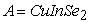) and B (e.g.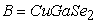), and the composition grading y(x) over the thickness of the layer: thus defining the composition values y at the back contact and junction of CIGS, and by specifying some grading law in between. The bandgap Eg of the material is then derived from the local composition parameter y(x), that is, Eg[y(x)] is evaluated. In this study, we used a parabolic grading law to define the local composition y(x) on the absorbing layer. The bandgap Eg is modeled according to a linear dependence of the local composition y(x). The dependence of Eg according to y(x) can be in the following equation form: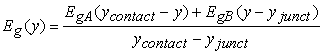(1)

With: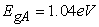and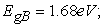respectively designate the bandgap of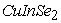and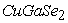.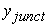and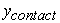designate, respectively, the local gallium compositions at the junction and the back contact of the CIGS absorber layer.

As previously announced, the local composition y(x), which represents the gallium content as a function of the depth x of the absorber layer, is modeled according to a parabolic grading law. This law may be in the following equation form: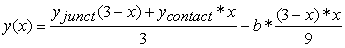(2)

This parabolic law y(x) is designed with three basic parameters:

• Definitions of the gallium level at the terminals of the absorber layer yjonct and ycontact ;

• The b parameter (bowing factor) which is used to modify volume distribution by playing on the opening and the offset of the parabola profile.

Thus, let's proceed to a series of variation of these parameters in order to study their different impact. These results are presented in the following paragraph.

3. Results and Discussions

3.1. Effect of the Bowing Factor (b)

In this first part, we studied three samples with which the bowing factor b takes the values 10%, 20% and 30%. These three samples were all modeled with a gallium level set at 30% at the junction and back contact of the absorber layer. Thus, through these three samples, we study the impact of this factor b on the performance of the solar cell. Figure 1 below shows the effect of the b parameter on the external quantum efficiency.

We first notice in Figure 1 the different areas of absorption of the photocell. Indeed we observe a first peak around 350 nm, corresponding to the absorption threshold of the n-ZnS window layer. Then, a second peak is noticed around 530 nm corresponding to the n-CdS absorption threshold. And finally we have the third absorption zone which is that of the absorbing layer p-CIGS. In passing a third peak nearing the 100% is noticed at about 600 nm which corresponds to the optimal absorption wavelength of the CIGS. We will focus our study on the variations that take place in the CIGS since the difference of the samples used is in this place. Then the results obtained show a double impact of the factor b on the quantum efficiency of the cell. Indeed, we have noticed that a weak bowing factor is more favorable to the absorption of more energetic photons. This is clearly illustrated on the first magnification (green framework). Then we notice an inversion for the absorption of less energetic photons. That is, the decrease in factor b is less favorable to the absorption of these photons (illustrated on the second magnification). Figure 2 and Figure 3 show respectively the evolution of the recombination current density as a function of the bias voltage and also the variation of the generation current density as a function of the bowing factor b.

• Figure 1. Impact of the bowing factor on external quantum efficiency
• Figure 2. Influence of the bowing factor on the characteristic JTot_rec/V
• Figure 3. Influence of the bowing factor on the generation current density of the cell

We note in Figure 2 that the recombination current density of the three samples varies little with a bias voltage less than 0.6 V. However, the curve representing the sample having a factor b equal to 10% is detached from the batch by providing a lower recombination current compared to the other two samples. Beyond this voltage, we notice a rise in recombination current densities for the three samples. The sample with factor b equal to 30% exhibits the greatest increase in recombination current relative to the other samples. This increase can be attributed to the accumulation of intrinsic defects in the material as the bowing of the parabolic profile of the gap increases. Indeed, strong curvatures can act as an electron barrier and thus as a source of recombination 5. Also, in Figure 3, we observe an increase in the generation current density as a function of this parameter b. Which is logical since the increase of this parameter widens the absorption range of the incident photons (see Figure 3). However, this increase would be positive if the recombination current did not follow the same allure. Thus, according to Figure 2, although there is a greater generation of carriers when flexion increases, the risk of these carriers recombining before collection becomes much greater. Figure 4 and Figure 5 show respectively the characteristics J/V and P/V of these three samples.

• Figure 4. Influence of the bowing factor on the J/V characteristic
• Figure 5. Influence of the bowing factor on the P/V characteristic

The current density collected for the various samples is substantially the same when the bias voltage is less than 0.6 V. This is hardly surprising since the recombination current density is almost the same in this interval. However, beyond this voltage, the responses recorded with the samples are distinguished. This is mainly due to the increase in the dark current density of the samples which is caused by the accumulation of intrinsic defects in the material. This difference is more visible in the vicinity of the maximum power point (MPP) shown in Figure 5. Indeed we notice that the sample with the lowest factor b (10%) provides a better power density at the optimal operating point. The variations of the macroscopic electrical parameters as a function of the bowing factor b are presented in Table 1 below.

We note in Table 1 that the increase of "b" has antagonistic effects on the open circuit voltage (Voc) and the short circuit current density (Jsc). Indeed, there is a clear decrease as the bowing of the parabolic profile of the gap becomes more pronounced. And also, conversely, the short-circuit current density takes an ascending allure when this factor increases. The effects of variation are not limited to Voc and Jsc. Its effect also affects the fill factor (FF) of the cell and therefore the conversion efficiency (η). We found that increasing "b" decreases the fill factor and conversion efficiency of the photocell. This confirms the results presented previously and also supports the hypothesis that the increase of this parameter contributes to the increase of defects intrinsic to the material; therefore decreases the performance of the cell.

3.2. Effect of Gallium Ratio ycontact at Back Contact

In this second part, we will study five samples to which the gallium content is varied between 25% and 35% at the back contact. The other parameters of the parabolic bandgap profile such as the bowing factor or the gallium content at the junction were set at 10% and 30%, respectively. We will use the results obtained with the five samples to determine the effect of the gallium, ycontact, ratio variation on the solar cell performance. In Figure 6 below is presented the external quantum efficiency obtained with the five samples.

The variation of the gallium content at the back contact of the absorber layer affects the external quantum efficiency of the cell. Indeed, we notice that the increase of ycontact is favorable to the absorption of photons at long wavelengths. This improvement of the quantum efficiency, due to the increase of the gallium content in the back contact, can be explained by the dependence of the bandgap at the rate of gallium. Indeed, according to the equation 1, the effective bandgap of the CIGS material increases as the gallium content increases in back contact. This bandgap grading, favored by the increase of the back bandgap, has the effect of fighting against the heating due to the absorption of very energetic photons. This justifies the improvement observed on quantum efficiency. We will find in Figure 7 and Figure 8 the evolution of the generation current density and the characteristic Jtot_rec/V depending on the gallium rate at the back contact.

• Figure 6. Impact of ycontact rate on external quantum efficiency
• Figure 7. Influence of the gallium rate ycontact on the generation current density
• Figure 8. Influence of the gallium rate ycontact on the JTot_rec/V characteristic

We notice on Figure 7 a decrease in the density of the generation current as the ycontact ratio increases. This decrease is mainly related to the increase of the back bandgap of the CIGS absorber. Indeed, when the bandgap is smaller, we are witnessing an increase in the generation of electron-hole pairs, thus to the increase of the generation current noticed. However, a smaller bandgap at the back contact tends to favor increased recombination in this area 6. This effect is perfectly illustrated in Figure 8. Due to the small bandgap at the back contact (relative to that at the junction), the induced potential difference is opposed to the transport of electrons to the space charge area. Hence the increase in the recombination rate. On the other hand, when the bandgap at the back contact is greater than at the junction, the transport of the electrons towards the space charge zone is facilitated so the collection rate increases and there is less recombination 7. Figure 9 and Figure 10 show respectively the characteristics of the output current and power density as a function of the bias voltage for the five samples studied.

• Figure 9. Influence of the gallium rate ycontact on the J/V characteristic
• Figure 10. Influence of the gallium rate ycontact on the P/V characteristic

We note in Figure 9 that the increase of the gallium content in the back contact of the absorber layer enhances the electrical performance of the cell. The reduction of the recombination current, when the increase of the Ga rate (shown in Figure 8) to the back face, therefore decreases the dark current and thus causes the increase of the collected current density. Indeed, we have confirmation of this improvement in Figure 10 which highlights its effect at the optimal operating point of the cell. We can then say that the maximum power point of the solar cell is significantly improved when the rear gap increases. Also other parameters like Voc or Jsc are improved at the same time. This effect of the variation of the back bandgap on the macroscopic electrical parameters of the photocell is clearly illustrated in Table 2 which show the fluctuations of the short circuit current density (Jsc), of the open circuit voltage (Voc), the fill factor (FF) and conversion efficiency (η) of the solar cell as a function of the Ga content in the back contact.

In Table 2, we notice an increasing evolution of the open circuit voltage (Vco) and also of the short circuit current density (Jsc) when the back bandgap increases. Which could be predictable with the Voc since it is intrinsically linked to the effective bandgap of the material and also that the recombinations are reduced during this addition 8. We also note significant improvements in the fill factor (FF) and conversion efficiency (η) when increasing the back bandgap. We can therefore conclude this sub-part by saying that the increase of the back bandgap is very favorable to the improvement of the performances of the solar cell.

3.3. Effect of Gallium ratio yjunct at the Junction

In this last part, we set the parameters of the bandgap profile as the bowing factor at 10%, the Ga content at the back contact of the absorber at 35% and then we vary the Ga content at the junction between 25% and 35%. As a result, we will use five samples with Ga-specific ratio values at the junction (yjunct). The results of this study are presented below. First, the effect of yjunct variation on the external quantum efficiency of the cell is shown in Figure 11.

• Figure 11. Impact of yjunct rate on external quantum efficiency

The variation of Ga content at the junction shows a large effect on the absorptivity of the cell. Indeed, since the junction is at the heart of the space charge zone, the fact of changing the bandgap at this level could only be felt. We notice on Figure 11 that the reduction of Ga at the junction is favorable to the absorptivity of the material. And yes, according to equation 1, the decrease of yjunct, leads to the increase of the effective bandgap of the material; therefore to the widening of the absorption range of the incident photons. We note that the sample with the lowest gallium content at the junction has higher photon absorptivity. This was predictable since the material mainly absorbs only photons having an energy greater than or equal to that of the bandgap. Then with the decrease of the yjunct content, we create a graded bandgap profile ascending from the junction to the back contact of the CIGS absorber layer. It is this graded bandgap that favors the absorption of photons over a longer wavelength range (observed in Figure 11). We present respectively in Figure 12 and Figure 13 the influence of the gallium yjunct rate on the characteristic JTot_rec/V and the generation current density for the various samples used.

• Figure 12. Influence of the gallium rate yjunct on the JTot_rec/V characteristic
• Figure 13. Influence of the gallium rate yjunct on the generation current density

We notice on Figure 12 (for a bias voltage between 0 and 0.65 V) the recombination current density is lower for samples with a lower Ga content at the junction. However, beyond 0.65 V, we notice an inversion of the evolution that is to say the sample with the lowest rate yjunct provides a larger recombination current which takes an exponential allure. Indeed, this phenomenon can be explained by the fact that when the junction is forward biased, the potential barrier decreases as the applied voltage increases. This gives a logical explanation for this phenomenon since the sample with the lowest yjunct rate also has the smallest potential barrier. So it is the collapse first of the latter which thus promotes the exponential increase of the recombination current. Also on Figure 13, we notice a decrease in carrier current density when the Ga content increases at the junction. This degradation of the generation current is the result of the increase of the bandgap at the junction of the absorbing layer which is intrinsically related to the yjunct rate. Figure 14 and Figure 15 show respectively the characteristics of the output current and power density as a function of the bias voltage. These figs illustrate the effect of the variation of the yjunct rate on the electrical performance of the photocell.

• Figure 14. Influence of the gallium rate yjunct on the J/V characteristic
• Figure 15. Influence of the gallium rate yjunct on the P/V characteristic

We notice on Figure 14 a decrease in the current density collected when the gallium content increases at the junction. This observed degradation can be explained by the fact that the dark current density, precisely that of recombination, is lower when the yjunct ratio is lower (Figure 12), and also by the fact that the generation current density is larger at this level (Figure 13). We have confirmation of this improvement in power density collected in Figure 15. This improvement is most noticeable at the maximum power point of the cell (MPP) which thus testifies to the improvement of the electrical quality of the material. In Table 3 is presented the variations of the macroscopic electrical parameters of the cell during the variation of the Ga ratio yjunct at the junction.

We observe as seen previously a clear improvement of the macroscopic electrical parameters of the cell when the yjunct rate at the junction is less. Indeed, the general remark is that only the open circuit voltage (Voc) increases as the Ga content increases at the junction. This is mainly due to the increase of the effective bandgap of the absorber layer. On the other hand, we also observe an improvement of the open-circuit current density (Jsc), of the fill factor (FF) and therefore of the conversion efficiency (η) of the cell when the ratio yjunct is at 25%.

4. Conclusion

This simulation allowed the study of different samples of photovoltaic cells based on CIGS. Our objective was to gain knowledge about the effect of bandgap geometry and adjustments for improved conversion efficiency (η). The results obtained clearly showed that the photovoltaic characteristics are very affected by the variation of the band gap geometry. Thus, after a few adjustments with respect to the gallium composition, we obtained a sample with a conversion efficiency of around 23.68%.

References

  CIGS solar cells: 22.6% conversion efficiency at ZSW, [online] “L’écho du solaire”. [Online]. Available: http://www.lechodusolaire.fr/cellules-solaires-cigs-226-de-rendement-de-conversion-zsw/#lightbox/1/[Accessed Feb. 12, 2018]. In article View Article  R. Herberholz, M. Igalson, and H. W. Schock, “Distinction of bulk and interface states in CuInSe2/CdS/ZnO by space charge spectroscopy” Journal of Applied Physics, 83 (01), pp. 318, 1998. In article View Article  M. Burgelman, P. Nollet and S. Degrave. “Modelling Polycrystalline semiconductor solar cells” Thin Solid Films, 361-362, pp. 527-532, 2000. In article View Article  M. Burgelman, J. Marlein, “Analysis of graded band gap solar cells with SCAPS”, Proceedings of the 23rd European Photovoltaic Solar Energy Conference, 1-5 September 2008, Valencia, Spain, pp. 2151-2155. In article View Article  A. Chirila, S. Buecheler, F. Pianezzi, P. Bloesch, C. Gretener, A. R. Uhl, C. Fella, L. Kranz, J. Perrenoud, S. Seyrling, R. Verma, S. Nishiwaki, Y. E. Romanyuk, G. Bilger and A. N. Tiwari, “Highly efﬁcient Cu(In,Ga)Se 2 solar cells grown on ﬂexible polymer ﬁlms”, Nature materials, 10 (11): 857-861, 2011. In article View Article  PubMed  Marianna Kemell, Mikko Ritala, and Markku Leskelä. “Thin Film Deposition Methods for CuInSe2 Solar Cells”, Critical Reviews in Solid State and Materials Sciences, 30 (1): 1-31, 2005. In article View Article  M. Turcu, I. M. Kotschau, and U. Rau. “Composition dependence of defect energies and band alignments in the Cu(In,Ga)(Se,S)2 alloy system” Journal of Applied Physics, 91 (3): 1391, 2002. In article View Article  T. Nakada, Invited Paper: “CIGS-based thin film solar cells and modules: Unique material properties”, Electronic Materials Letters, 8 (2): 179-185, 2012. In article View Article

Published with license by Science and Education Publishing, Copyright © 2018 Ousmane Diagne, Djimba Niane, Alain Kassine Ehemba, Mouhamadou Mamour Soce and Moustapha DiengThis work is licensed under a Creative Commons Attribution 4.0 International License. To view a copy of this license, visit http://creativecommons.org/licenses/by/4.0/